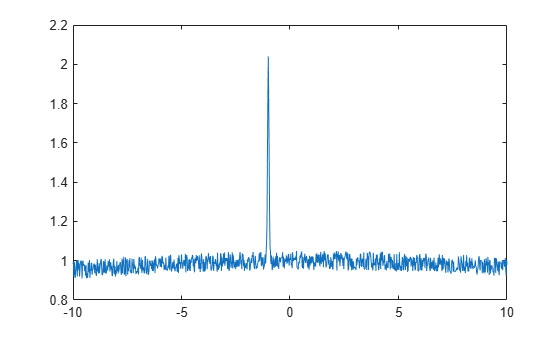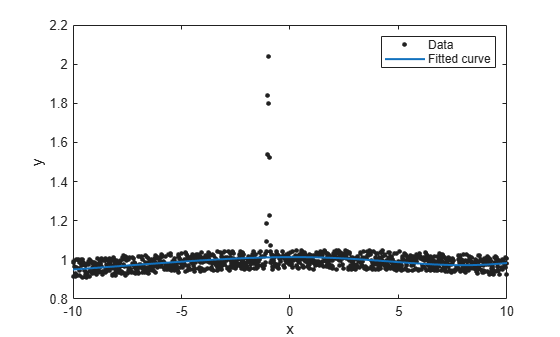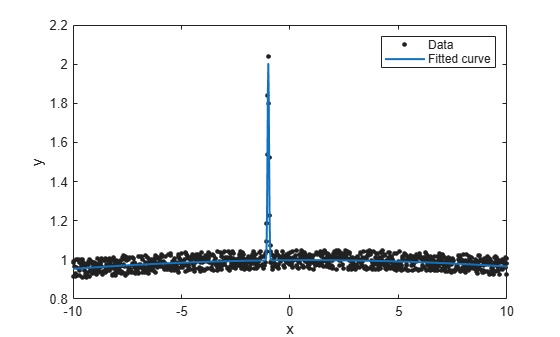# fitoptions

Create or modify fit options object

## Syntax

``fitOptions = fitoptions``
``fitOptions = fitoptions(libraryModelName)``
``fitOptions = fitoptions(libraryModelName,Name,Value)``
``fitOptions = fitoptions(fitType)``
``fitOptions = fitoptions(Name,Value)``
``newOptions = fitoptions(fitOptions,Name,Value)``
``newOptions = fitoptions(options1,options2)``

## Description

example

````fitOptions = fitoptions` creates the default fit options object `fitOptions`.```

example

````fitOptions = fitoptions(libraryModelName)` creates the default fit options object for the library model.```

example

````fitOptions = fitoptions(libraryModelName,Name,Value)` creates fit options for the specified library model with additional options specified by one or more `Name,Value` pair arguments.```

example

````fitOptions = fitoptions(fitType)` gets the fit options object for the specified `fitType`. Use this syntax to work with fit options for custom models.```

example

````fitOptions = fitoptions(Name,Value)` creates fit options with additional options specified by one or more `Name,Value` pair arguments.```

example

````newOptions = fitoptions(fitOptions,Name,Value)` modifies the existing fit options object `fitOptions` and returns updated fit options in `newOptions` with new options specified by one or more `Name,Value` pair arguments.```

example

````newOptions = fitoptions(options1,options2)` combines the existing fit options objects `options1` and `options2` in `newOptions`. If `Method` agrees, the nonempty values for the properties in `options2` override the corresponding values in `options1` in `newOptions`.If `Method` differs, `newOptions` contains the `options1` value for `Method` and values from `options2` for `Normalize`, `Exclude`, and `Weights`. ```

## Examples

collapse all

Create the default fit options object and set the option to center and scale the data before fitting.

```options = fitoptions; options.Normal = 'on'```
```options = Normalize: 'on' Exclude: [1x0 double] Weights: [1x0 double] Method: 'None' ```
`options = fitoptions('gauss2')`
```options = Normalize: 'off' Exclude: [] Weights: [] Method: 'NonlinearLeastSquares' Robust: 'Off' StartPoint: [1x0 double] Lower: [-Inf -Inf 0 -Inf -Inf 0] Upper: [1x0 double] Algorithm: 'Trust-Region' DiffMinChange: 1.0000e-08 DiffMaxChange: 0.1000 Display: 'Notify' MaxFunEvals: 600 MaxIter: 400 TolFun: 1.0000e-06 TolX: 1.0000e-06 ```

Create fit options for a cubic polynomial and set center and scale and robust fitting options.

`options = fitoptions('poly3', 'Normalize', 'on', 'Robust', 'Bisquare')`
```options = Normalize: 'on' Exclude: [] Weights: [] Method: 'LinearLeastSquares' Robust: 'Bisquare' Lower: [1x0 double] Upper: [1x0 double] ```
`options = fitoptions('Method', 'LinearLeastSquares')`
```options = Normalize: 'off' Exclude: [] Weights: [] Method: 'LinearLeastSquares' Robust: 'Off' Lower: [1x0 double] Upper: [1x0 double] ```

Modifying the default fit options object is useful when you want to set the `Normalize`, `Exclude`, or `Weights` properties, and then fit your data using the same options with different fitting methods. For example, the following uses the same fit options to fit different library model types.

```load census options = fitoptions; options.Normalize = 'on'; f1 = fit(cdate,pop,'poly3',options); f2 = fit(cdate,pop,'exp1',options); f3 = fit(cdate,pop,'cubicspline',options)```
```f3 = Cubic interpolating spline: f3(x) = piecewise polynomial computed from p where x is normalized by mean 1890 and std 62.05 Coefficients: p = coefficient structure ```

Find the smoothing parameter. Data-dependent fit options such as the `smooth` parameter are returned in the third output argument of the `fit` function.

```load census [f,gof,out] = fit(cdate,pop,'SmoothingSpline'); smoothparam = out.p```
```smoothparam = 0.0089 ```

Modify the default smoothing parameter for a new fit.

```options = fitoptions('Method','SmoothingSpline',... 'SmoothingParam',0.0098); [f,gof,out] = fit(cdate,pop,'SmoothingSpline',options);```

Create a Gaussian fit, inspect the confidence intervals, and specify lower bound fit options to help the algorithm.

Create a noisy sum of two Gaussian peaks, one with a small width, and one with a large width.

```a1 = 1; b1 = -1; c1 = 0.05; a2 = 1; b2 = 1; c2 = 50; x = (-10:0.02:10)'; gdata = a1*exp(-((x-b1)/c1).^2) + ... a2*exp(-((x-b2)/c2).^2) + ... 0.1*(rand(size(x))-.5); plot(x,gdata)```Fit the data using the two-term Gaussian library model.

`gfit = fit(x,gdata,'gauss2') `
```gfit = General model Gauss2: gfit(x) = a1*exp(-((x-b1)/c1)^2) + a2*exp(-((x-b2)/c2)^2) Coefficients (with 95% confidence bounds): a1 = -0.1451 (-1.485, 1.195) b1 = 9.725 (-14.7, 34.15) c1 = 7.117 (-15.84, 30.07) a2 = 14.08 (-1.962e+04, 1.965e+04) b2 = 607.4 (-3.197e+05, 3.209e+05) c2 = 376 (-9.745e+04, 9.82e+04) ```
`plot(gfit,x,gdata)`The algorithm is having difficulty, as indicated by the wide confidence intervals for several coefficients.

To help the algorithm, specify lower bounds for the nonnegative amplitudes `a1` and `a2` and widths `c1`, `c2`.

`options = fitoptions('gauss2', 'Lower', [0 -Inf 0 0 -Inf 0]);`

Alternatively, you can set properties of the fit options using the form `options.Property = NewPropertyValue`.

```options = fitoptions('gauss2'); options.Lower = [0 -Inf 0 0 -Inf 0];```

Recompute the fit using the bound constraints on the coefficients.

`gfit = fit(x,gdata,'gauss2',options) `
```gfit = General model Gauss2: gfit(x) = a1*exp(-((x-b1)/c1)^2) + a2*exp(-((x-b2)/c2)^2) Coefficients (with 95% confidence bounds): a1 = 1.005 (0.966, 1.044) b1 = -1 (-1.002, -0.9988) c1 = 0.0491 (0.0469, 0.0513) a2 = 0.9985 (0.9958, 1.001) b2 = 0.8059 (0.3879, 1.224) c2 = 50.6 (46.68, 54.52) ```
`plot(gfit,x,gdata)`This is a much better fit. You can further improve the fit by assigning reasonable values to other properties in the fit options object.

Create fit options and set lower bounds.

`options = fitoptions('gauss2', 'Lower', [0 -Inf 0 0 -Inf 0])`
```options = Normalize: 'off' Exclude: [] Weights: [] Method: 'NonlinearLeastSquares' Robust: 'Off' StartPoint: [1x0 double] Lower: [0 -Inf 0 0 -Inf 0] Upper: [1x0 double] Algorithm: 'Trust-Region' DiffMinChange: 1.0000e-08 DiffMaxChange: 0.1000 Display: 'Notify' MaxFunEvals: 600 MaxIter: 400 TolFun: 1.0000e-06 TolX: 1.0000e-06 ```

Make a new copy of the fit options and modify the robust parameter.

`newoptions = fitoptions(options, 'Robust','Bisquare')`
```newoptions = Normalize: 'off' Exclude: [] Weights: [] Method: 'NonlinearLeastSquares' Robust: 'Bisquare' StartPoint: [1x0 double] Lower: [0 -Inf 0 0 -Inf 0] Upper: [1x0 double] Algorithm: 'Trust-Region' DiffMinChange: 1.0000e-08 DiffMaxChange: 0.1000 Display: 'Notify' MaxFunEvals: 600 MaxIter: 400 TolFun: 1.0000e-06 TolX: 1.0000e-06 ```

Combine fit options.

`options2 = fitoptions(options, newoptions)`
```options2 = Normalize: 'off' Exclude: [] Weights: [] Method: 'NonlinearLeastSquares' Robust: 'Bisquare' StartPoint: [1x0 double] Lower: [0 -Inf 0 0 -Inf 0] Upper: [1x0 double] Algorithm: 'Trust-Region' DiffMinChange: 1.0000e-08 DiffMaxChange: 0.1000 Display: 'Notify' MaxFunEvals: 600 MaxIter: 400 TolFun: 1.0000e-06 TolX: 1.0000e-06 ```

Create a linear model fit type.

`lft = fittype({'x','sin(x)','1'})`
```lft = Linear model: lft(a,b,c,x) = a*x + b*sin(x) + c ```

Get the fit options for the fit type `lft`.

`fo = fitoptions(lft)`
```fo = Normalize: 'off' Exclude: [] Weights: [] Method: 'LinearLeastSquares' Robust: 'Off' Lower: [1x0 double] Upper: [1x0 double] ```

Set the normalize fit option.

`fo.Normalize = 'on'`
```fo = Normalize: 'on' Exclude: [] Weights: [] Method: 'LinearLeastSquares' Robust: 'Off' Lower: [1x0 double] Upper: [1x0 double] ```

## Input Arguments

collapse all

Library model to fit, specified as a character vector or string scalar. This table shows some common examples.

Library Model Name

Description

`'poly1'`

Linear polynomial curve

`'poly11'`

Linear polynomial surface

`'poly2'`

`'linearinterp'`

Piecewise linear interpolation

`'cubicinterp'`

Piecewise cubic interpolation

`'smoothingspline'`

Smoothing spline (curve)

`'lowess'`

Local linear regression (surface)

For a list of library model names, see Model Names and Equations.

Example: `'poly2'`

Data Types: `char` | `string`

Model type to fit, specified as a `fittype` constructed with the `fittype` function. Use this to work with fit options for custom models.

Algorithm options, specified as a `fitoptions` object created using the `fitoptions` function.

Algorithm options to combine, constructed using the `fitoptions` function.

Algorithm options to combine, constructed using the `fitoptions` function.

### Name-Value Arguments

Specify optional pairs of arguments as `Name1=Value1,...,NameN=ValueN`, where `Name` is the argument name and `Value` is the corresponding value. Name-value arguments must appear after other arguments, but the order of the pairs does not matter.

Before R2021a, use commas to separate each name and value, and enclose `Name` in quotes.

Example: ```'Method','NonlinearLeastSquares','Lower',[0,0],'Upper',[Inf,max(x)],'Startpoint',[1 1]``` specifies fitting method, bounds, and start points.

Options for All Fitting Methods

collapse all

Option to center and scale the data, specified as the comma-separated pair consisting of `'Normalize'` and `'on'` or `'off'`.

Data Types: `char`

Points to exclude from the fit, specified as the comma-separated pair consisting of `'Exclude'` and one of:

• An expression describing a logical vector, e.g., `x > 10`.

• A vector of integers indexing the points you want to exclude, e.g., `[1 10 25]`.

• A logical vector for all data points where `true` represents an outlier, created by `excludedata`.

For examples, see `fit`.

Weights for the fit, specified as the comma-separated pair consisting of `'Weights'` and a vector the same size as number of data points.

Data Types: `double`

Fitting method, specified as the comma-separated pair consisting of `'Method'` and one of the fitting methods in this table.

Fitting Method

Description

`'NearestInterpolant'`

Nearest neighbor interpolation

`'LinearInterpolant'`

Linear interpolation

`'PchipInterpolant'`

Piecewise cubic Hermite interpolation (curves only)

`'CubicSplineInterpolant'`

Cubic spline interpolation

`'BiharmonicInterpolant'`

Biharmonic surface interpolation

`'SmoothingSpline'`

Smoothing spline

`'LowessFit'`

Lowess smoothing (surfaces only)

`'LinearLeastSquares'`

Linear least squares

`'NonlinearLeastSquares'`

Nonlinear least squares

Data Types: `char` | `string`

Smoothing Options

collapse all

Smoothing parameter, specified as the comma-separated pair consisting of `'SmoothingParam'` and a scalar value between 0 and 1. The default value depends on the data set. Only available if the `Method` is `SmoothingSpline`.

Data Types: `double`

Proportion of data points to use in local regressions, specified as the comma-separated pair consisting of `'Span'` and a scalar value between 0 and 1. Only available if the `Method` is `LowessFit`.

Data Types: `double`

Linear and Nonlinear Least-Squares Options

collapse all

Robust linear least-squares fitting method, specified as the comma-separated pair consisting of `'Robust'` and one of these values:

• `'LAR'` specifies the least absolute residual method.

• `'Bisquare'` specifies the bisquare weights method.

Available when the `Method` is `LinearLeastSquares` or `NonlinearLeastSquares`.

Data Types: `char`

Lower bounds on the coefficients to be fitted, specified as the comma-separated pair consisting of `'Lower'` and a vector. The default value is an empty vector, indicating that the fit is unconstrained by lower bounds. If bounds are specified, the vector length must equal the number of coefficients. Find the order of the entries for coefficients in the vector value by using the `coeffnames` function. For an example, see `fit`. Individual unconstrained lower bounds can be specified by `-Inf`.

Available when the `Method` is `LinearLeastSquares` or `NonlinearLeastSquares`.

Data Types: `double`

Upper bounds on the coefficients to be fitted, specified as the comma-separated pair consisting of `'Upper'` and a vector. The default value is an empty vector, indicating that the fit is unconstrained by upper bounds. If bounds are specified, the vector length must equal the number of coefficients. Find the order of the entries for coefficients in the vector value by using the `coeffnames` function. For an example, see `fit`. Individual unconstrained upper bounds can be specified by `+Inf`.

Available when the `Method` is `LinearLeastSquares` or `NonlinearLeastSquares`.

Data Types: `logical`

Nonlinear Least-Squares Options

collapse all

Initial values for the coefficients, specified as the comma-separated pair consisting of `'StartPoint'` and a vector. Find the order of the entries for coefficients in the vector value by using the `coeffnames` function. For an example, see `fit`.

If no start points (the default value of an empty vector) are passed to the `fit` function, starting points for some library models are determined heuristically. For rational and Weibull models, and all custom nonlinear models, the toolbox selects default initial values for coefficients uniformly at random from the interval (0,1). As a result, multiple fits using the same data and model might lead to different fitted coefficients. To avoid this, specify initial values for coefficients with a vector value for the `StartPoint` property.

Available when the `Method` is `NonlinearLeastSquares`.

Data Types: `double`

Algorithm to use for the fitting procedure, specified as the comma-separated pair consisting of `'Algorithm'` and either `'Levenberg-Marquardt'` or `'Trust-Region'`.

Available when the `Method` is `NonlinearLeastSquares`.

Data Types: `char`

Maximum change in coefficients for finite difference gradients, specified as the comma-separated pair consisting of `'DiffMaxChange'` and a scalar.

Available when the `Method` is `NonlinearLeastSquares`.

Data Types: `double`

Minimum change in coefficients for finite difference gradients, specified as the comma-separated pair consisting of `'DiffMinChange'` and a scalar.

Available when the `Method` is `NonlinearLeastSquares`.

Data Types: `double`

Display option in the command window, specified as the comma-separated pair consisting of `'Display'` and one of these options:

• `'notify'` displays output only if the fit does not converge.

• `'final'` displays only the final output.

• `'iter'` displays output at each iteration.

• `'off'` displays no output.

Available when the `Method` is `NonlinearLeastSquares`.

Data Types: `char`

Maximum number of evaluations of the model allowed, specified as the comma-separated pair consisting of `'MaxFunEvals'` and a scalar.

Available when the `Method` is `NonlinearLeastSquares`.

Data Types: `double`

Maximum number of iterations allowed for the fit, specified as the comma-separated pair consisting of `'MaxIter'` and a scalar.

Available when the `Method` is `NonlinearLeastSquares`.

Data Types: `double`

Termination tolerance on the model value, specified as the comma-separated pair consisting of `'TolFun'` and a scalar.

Available when the `Method` is `NonlinearLeastSquares`.

Data Types: `double`

Termination tolerance on the coefficient values, specified as the comma-separated pair consisting of `'TolX'` and a scalar.

Available when the `Method` is `NonlinearLeastSquares`.

Data Types: `double`

## Output Arguments

collapse all

Algorithm options, returned as a `fitoptions` object.

New algorithm options, returned as a `fitoptions` object.

## Version History

Introduced before R2006a